+

# Output Optimization: Total Revenue / Total Cost

Author: Sophia Tutorial
##### Description:

Determine the relationship between total cost, total revenue, and profit.

(more)### Developing Effective Teams

*No strings attached. This college course is 100% free and is worth 1 semester credit.

37 Sophia partners guarantee credit transfer.

299 Institutions have accepted or given pre-approval for credit transfer.

* The American Council on Education's College Credit Recommendation Service (ACE Credit®) has evaluated and recommended college credit for 32 of Sophia’s online courses. Many different colleges and universities consider ACE CREDIT recommendations in determining the applicability to their course and degree programs.

Tutorial
what's covered
This tutorial will cover the topic of output optimization, focusing on total revenue and total cost.

Our discussion breaks down as follows:

1. Total Revenue, Total Cost, and Profit
2. Maximizing Profit
3. Maximizing Profit: Graph
1. Equating Slope of Total Revenue and Total Cost

## 1. Total Revenue, Total Cost, and Profit

Now, we know that the point of owning a business is to make a profit. We also know that profit equals revenues minus costs.

Profit = Revenues - Costs

In many tutorials, we focus on one or the other. However, in this tutorial, we will be looking at both revenue and costs, because we want to determine what is maximizing profit.

Total revenue is all sales generated by a firm from the sale of a product or service.

It is price times quantity, or everything that a business is selling times the price of that good.

formula
Total Revenue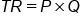Total cost is everything it costs to get that product to market, meaning all variable and fixed costs associated with production--including opportunity cost, remember, in economics.

formula
Total Cost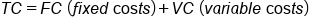Finally, we take our total revenue and subtract our total cost to arrive at profit.

formula
Accounting Profit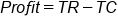terms to know
Total Revenue
All sales generated by a firm from the sale of a product or service
Total Cost
All variable and fixed costs associated with production which includes opportunity cost
Profit
Total revenue minus total cost

## 2. Maximizing Profit

Let's look at an example of perfect competition, where the price of the product each time is the same at \$20 per t-shirt.

hint
Because this is perfect competition, this company is a price-taker.

Number of T-Shirts Total Revenue Total Cost Profit
0 \$0 \$30 -\$30
1 \$20 \$35 -\$15
2 \$40 \$38 \$2
3 \$60 \$43 \$17
4 \$80 \$50 \$30
5 \$100 \$60 \$40
6 \$120 \$72 \$48
7 \$140 \$87 \$53
8 \$160 \$107 \$53
9 \$180 \$130 \$50
10 \$200 \$155 \$45

Notice the following in the calculations of the chart:

• Total revenue is the price times quantity, so it goes up by \$20 each time.
• Total cost is the fixed costs plus variable costs; these columns are not shown on the chart to keep it simple, but keep in mind that total cost would be fixed and variable added together.
• Profit is total revenue minus total cost. Notice in the beginning, profit is negative because they haven't produced anything yet, even though they had a fixed cost that they had to pay. It only starts to rise as they begin to produce more.

It is important to note that when looking at profit maximization, the goal is not to simply minimize total cost.

This is because minimizing costs would refer to that \$30 in the first row of the total cost column. However, that is not at all where profit is the highest, as mentioned.

The goal is also not to maximize total revenue. You may think that a firm should just crank out as much as possible, but notice that at some point--like the bottom row of the chart--profit actually begins to go back down.

Instead, we are looking at where profit is the highest.

You can see that there are two places where profit is the highest. It is where there is the greatest difference between total revenue and total cost.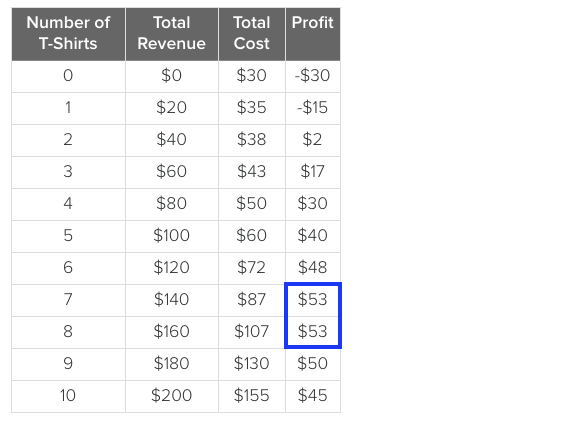Since profit is total revenue minus total cost, wherever these two are the greatest amount apart is where profit will be the highest.

## 3. Maximizing Profit: Graph

Let's look at maximizing profit on a graph.

Notice total revenue is the straight line because the price is \$20 each time. Total cost is the red curve, while profit is the green curve.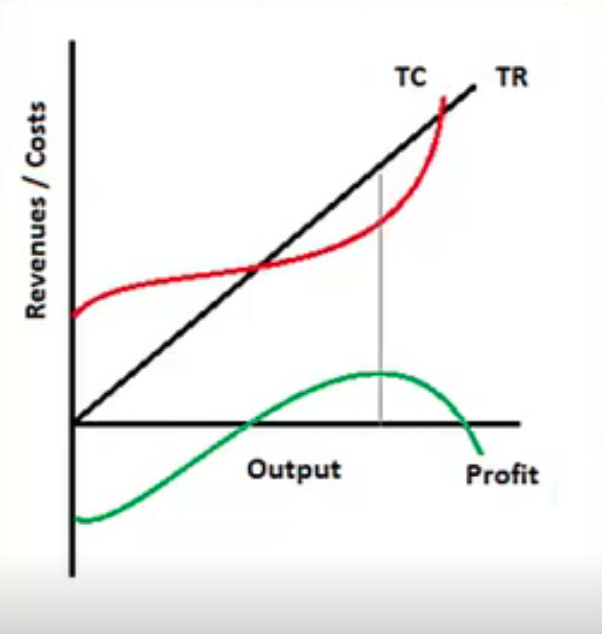Notice that profit was negative at first, then it eventually reaches its high point and begins to taper off again.

Profit is the highest where there is the biggest gap between total revenue and total cost, shown by the gray line.

3a. Equating Slope of Total Revenue and Total Cost

Note that this is also where the slope of total revenue and total cost are equal.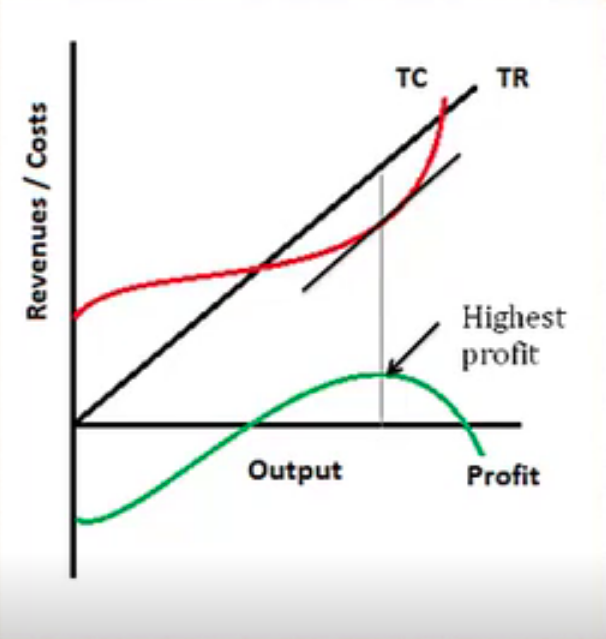At the point where the difference is the greatest, where profit is the highest, the slope of total cost is exactly the same as the slope of total revenue.

Why is that? Well, slope is rise over run. It is the change in the value of the y-axis variable relative to a change in the x-axis variable.

Therefore, we are looking at the rate of change of total revenue and the rate of change of total cost.

Now, if total revenue is steeper than total cost (shown below), this means that revenues are increasing faster than costs.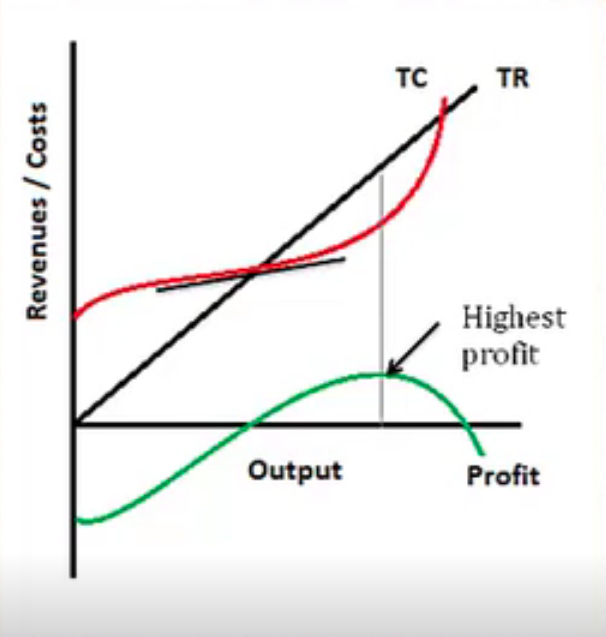Now, this is a good thing, but if we want to maximize profit, we need to produce more, because producing more means that we are going to add more to the revenue side than to the cost. This means we could actually do better by producing more.

On the other hand, if total cost is steeper than total revenue, this is the opposite situation.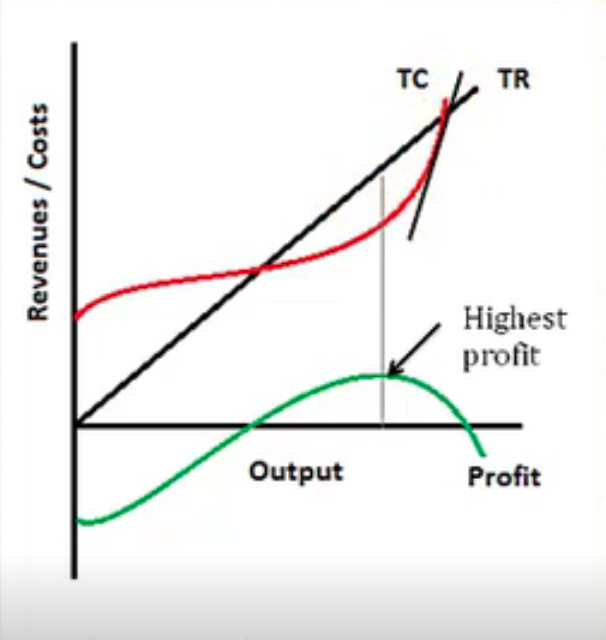This means that as we are producing more, we are adding more to cost, and costs are increasing faster than revenues--in which case, we need to scale back and produce less.

big idea
Therefore, profit will be highest when we produce to the point where the slope of total revenue and total cost are equal.

term to know
Slope
Rise over run; change in the value of the y-axis variable relative to a change in the x-axis variable

summary
We began today's lesson by reviewing total revenue, total cost, and profit. We learned that a firm will maximize profit where there is the greatest difference--or distance of a graph--between total revenue and total cost. We also learned that this will be where the slope of total revenue and total cost are equal.

Source: Adapted from Sophia instructor Kate Eskra.

Terms to Know
Profit

Total revenue minus total cost.

Slope

Rise over run; change in the value of the y-axis variable relative to a change in the x-axis variable.

Total Cost

All variable and fixed costs associated with production which includes opportunity cost.

Total Revenue

All sales generated by a firm from the sale of a product or service.

Formulas to Know
Accounting ProfitTotal CostTotal RevenueRating